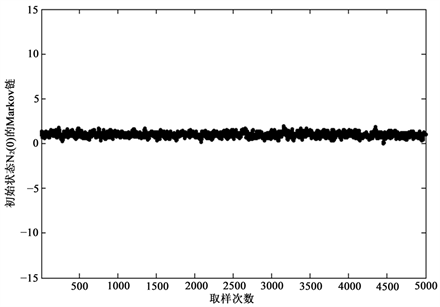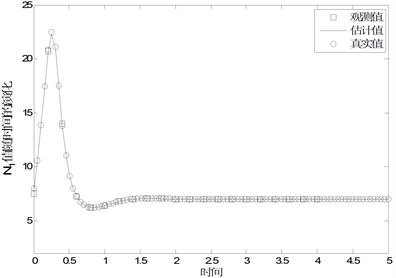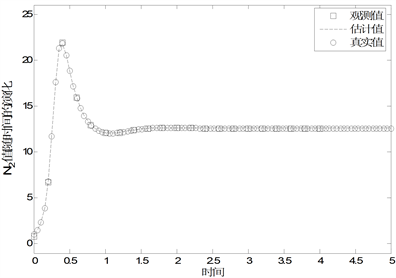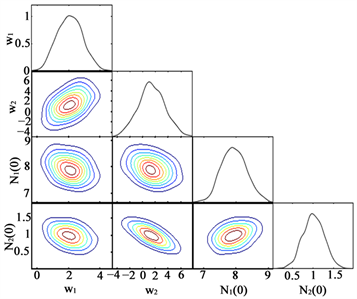﻿ 基于贝叶斯MCMC方法的资料同化技术研究

# 基于贝叶斯MCMC方法的资料同化技术研究Bayesian MCMC Method for the Solution of Data Assimilation

Abstract: In the framework of Bayesian theorem, a new method is proposed to find the solution of data as-similation problem based on Markov Chain Monte Carlo (MCMC) algorithm, which can quantify the posterior probability density function (PPDF) for initial states and model errors of nonlinear dy-namical system. Firstly, the PPDF for unknown initial states and model errors which are derived with the Bayesian method and parameters to be estimated can be thought as the mathematic ex-pectation of corresponding marginal PPDF. Secondly, taking the posterior probability as the inva-riant distribution, the Adaptive Metropolis algorithm is used to construct the Markov Chains of unknown initial states and model errors, respectively. That is, importance sampling of the posterior distribution is carried out. And the converged samples are used to calculate the mathematic expectation. So far, initial states and model errors of nonlinear dynamical system are estimated by Bayesian MCMC method successfully. Then, one and two-dimensional posterior distributions are constructed from the converged samples of initial states and model errors. And two-dimensional posterior distributions depict the interactions and correlations quantitatively between two dif-ferent and arbitrary parameters. Finally, the results of numerical experiments show that the new data assimilation method can estimate initial conditions of nonlinear dynamical system very con-veniently and accurately.

1. 引言

2. 贝叶斯理论

$\left\{\begin{array}{l}\stackrel{˙}{x}\left(t\right)=F\left[x\left(t\right),t\right]+w,\text{\hspace{0.17em}}\text{\hspace{0.17em}}\text{\hspace{0.17em}}t\in \left[0,T\right]\\ x|{}_{t=0}={x}_{0},\end{array}$

$p\left(m|y\right)=\frac{p\left(y|m\right)p\left(m\right)}{p\left(y\right)}$ (2)

$p\left(m|y\right)\propto p\left(y|m\right)p\left(m\right)$ (3)

(3)式是进行贝叶斯推理的基础，各项的物理或统计意义解释如下：先验概率分布 $p\left(m\right)$ 包含了观测获取前就存在的未知随机向量先验信息，它是在进行贝叶斯推理时的一种必要信息。从反问题的角度，先验信息的引入增加了信息容量、减小了不确定性范围，从而可以部分克服反问题不适定性。先验信息主要来源于历史观测资料、经验和主观判断等，例如：变分资料同化中的背景场及其误差协方差矩阵就是一种典型先验信息。条件概率密度 $p\left(y|m\right)$ 又称似然(Likelihood)函数，也可以写作 $L\left(y|m\right)$ ，包含了观测信息，即在有观测条件下未知参数的似然度信息。通俗地说，其表示了所估计的模式初始状态和模式误差与观测量之间的拟合程度，值越大表明拟合程度越好，反之越差。通过融合先验信息和观测信息后，就得到反映未知随机向量 $m$ 整体信息的后验概率密度函数 $p\left(m|y\right)$ ，它定义在初始状态和模式误差的整个解空间，表示问题的“完全”解。

3. MCMC算法

MCMC (Markov chain Monte Carlo)方法是现代统计计算中最重要的算法之一，通过MCMC方法可以得到复杂模型中众多物理参数的有效范围。MCMC算法基于马尔科夫链的采样机制，可以对定义在高维随机向量空间 $M$ 上无明确数学表达式的概率分布 $\pi$ 进行有效抽样，基本思想是产生大量服从分布 $\pi$ 的随机向量序列 $\left\{{m}_{\text{1}},{m}_{\text{2}},\cdots ,{m}_{I}\right\}$ ，其中I为抽样数。如果向量序列满足马尔可夫性质：向量 ${m}_{i+1}$ 的产生仅依赖于前一个向量 ${m}_{i}$ ，而与过去时刻 $i-1,i-2,\cdots ,1$ 的状态向量 ${m}_{i-1},{m}_{i-2},\cdots ,{m}_{1}$ 都无关，则该向量序列称为马尔可夫链。马尔可夫性质的另一种表述是：若抽样算法当前访问的是 ${m}_{j}$ 点，则下一步访问另一点 ${m}_{i}$ 的概率只依赖于 ${m}_{j}$ ，而与先前访问的点无关。马尔科夫性质意味着抽样算法完全可由转移概率矩阵 $\pi$ 描述，矩阵元素 ${\pi }_{ij}$ 表示算法在当前访问 ${m}_{j}$ 的条件下接着将要访问 ${m}_{i}$ 的条件概率。按照构造Markov链所用转移概率矩阵的不同，MCMC方法的主要抽样算法有：Gibbs抽样器算法、Metropolis-Hastings算法和自适应Metropolis算法  。

${C}_{i}=\left\{\begin{array}{l}{C}_{0},\text{\hspace{0.17em}}\text{\hspace{0.17em}}\text{\hspace{0.17em}}\text{\hspace{0.17em}}\text{\hspace{0.17em}}\text{\hspace{0.17em}}\text{\hspace{0.17em}}\text{\hspace{0.17em}}\text{\hspace{0.17em}}\text{\hspace{0.17em}}\text{\hspace{0.17em}}\text{\hspace{0.17em}}\text{\hspace{0.17em}}\text{\hspace{0.17em}}\text{\hspace{0.17em}}\text{\hspace{0.17em}}\text{\hspace{0.17em}}\text{\hspace{0.17em}}\text{\hspace{0.17em}}\text{\hspace{0.17em}}\text{\hspace{0.17em}}\text{\hspace{0.17em}}\text{\hspace{0.17em}}\text{\hspace{0.17em}}\text{\hspace{0.17em}}\text{\hspace{0.17em}}\text{\hspace{0.17em}}\text{\hspace{0.17em}}\text{\hspace{0.17em}}\text{\hspace{0.17em}}\text{\hspace{0.17em}}\text{\hspace{0.17em}}\text{ }\text{\hspace{0.17em}}\text{\hspace{0.17em}}\text{\hspace{0.17em}}\text{\hspace{0.17em}}\text{\hspace{0.17em}}i\le {i}_{0}\\ {s}_{d}Cov\left({\stackrel{^}{m}}_{0},\cdots ,{\stackrel{^}{m}}_{i-1}\right)+{s}_{d}\epsilon {I}_{d},\text{\hspace{0.17em}}\text{\hspace{0.17em}}\text{\hspace{0.17em}}i\ge {i}_{0}\end{array}$ (4)

${C}_{i+1}=\frac{i-1}{i}{C}_{i}+\frac{{s}_{d}}{i}\left(i{\stackrel{¯}{m}}_{i-1}{\stackrel{¯}{m}}_{i-1}^{\text{T}}-\left(i+1\right){\stackrel{¯}{m}}_{i}{\stackrel{¯}{m}}_{i}^{\text{T}}+{m}_{i}{m}_{i}^{\text{T}}+\epsilon {I}_{d}\right)$ (5)

1) 设定 $i=0$ ，对所有未知参数变量进行初始化；

2) 随机量的生成和接受，构造Markov链：

a) 利用公式(4)计算协方差矩阵 ${C}_{i}$

b) 产生服从高斯正态分布的推荐参数值 ${m}^{\ast }~N\left({m}_{i},{C}_{i}\right)$

c)计算接受概率 $\alpha =\mathrm{min}\left\{1,\frac{p\left(d|{m}^{\ast }\right)p\left({m}^{\ast }\right)}{p\left(d|{m}_{i}\right)p\left({m}_{i}\right)}\right\}$

d) 产生服从均匀分布的随机数 $u~U\left(0,1\right)$

e) 若 $u<\alpha$ ，则接受 ${m}_{i+1}={m}^{\ast }$ ，否则 ${m}_{i+1}={m}_{i}$

3) 重复上面的步骤(a)~(e)，直到产生预先指定数量的样本为止。

4. 资料同化问题求解

$\left\{\begin{array}{l}\text{d}{N}_{1}/\text{d}t=a{N}_{1}-c{N}_{1}{N}_{2}+{w}_{1},\\ \text{d}{N}_{2}/\text{d}t=b{N}_{2}+d{N}_{1}{N}_{2}-e{N}_{2}^{2}+{w}_{2},\\ \left({N}_{1},{N}_{2}\right)|{}_{t=0}=\left({N}_{1}\left(0\right),{N}_{2}\left(0\right)\right),\end{array}$ (6)

$\begin{array}{l}p\left[{N}_{1}\left(0\right),{N}_{2}\left(0\right),{w}_{1},{w}_{2}|y\right]\\ =p\left[y|{N}_{1}\left(0\right),{N}_{2}\left(0\right),{w}_{1},{w}_{2}\right]p\left[{N}_{1}\left(0\right),{N}_{2}\left(0\right),{w}_{1},{w}_{2}\right]\end{array}$ (7)

$L\left(y|m\right)=L\left(y|{x}_{0},w\right)=\frac{1}{{\left(2\text{π}{\sigma }_{o}^{2}\right)}^{n/2}}\mathrm{exp}\left[-{‖y-M\left({x}_{0},w\right)‖}^{2}/\left(2{\sigma }_{o}^{2}\right)\right]$ (8)

$p\left(m\right)=U\left[{N}_{1}\left(0\right)\right]U\left[{N}_{2}\left(0\right)\right]U\left({w}_{1}\right)U\left({w}_{2}\right)$ (9)

$p\left({x}_{0},w|y\right)=\frac{1}{{\left(2\text{π}{\sigma }_{o}^{2}\right)}^{n/2}}\mathrm{exp}\left[-\frac{{‖y-M\left({x}_{0},w\right)‖}^{2}}{2{\sigma }_{o}^{2}}\right]U\left[{N}_{1}\left(0\right)\right]U\left[{N}_{2}\left(0\right)\right]U\left({w}_{1}\right)U\left({w}_{2}\right)$ (10)

5. 数值试验结果

$U\left[{N}_{1}\left(0\right)\right]=\left\{\begin{array}{l}1/30\text{\hspace{0.17em}}\text{\hspace{0.17em}}\text{\hspace{0.17em}}\text{\hspace{0.17em}}\text{\hspace{0.17em}}-15<{N}_{1}\left(0\right)<15\\ 0\text{\hspace{0.17em}}\text{\hspace{0.17em}}\text{\hspace{0.17em}}\text{\hspace{0.17em}}\text{\hspace{0.17em}}\text{\hspace{0.17em}}\text{\hspace{0.17em}}\text{\hspace{0.17em}}\text{\hspace{0.17em}}\text{\hspace{0.17em}}\text{\hspace{0.17em}}\text{\hspace{0.17em}}\text{\hspace{0.17em}}\text{\hspace{0.17em}}otherwise\end{array}$(11)

$U\left[{N}_{2}\left(0\right)\right]=\left\{\begin{array}{l}1/30\text{\hspace{0.17em}}\text{\hspace{0.17em}}\text{\hspace{0.17em}}\text{\hspace{0.17em}}\text{\hspace{0.17em}}\text{\hspace{0.17em}}\text{\hspace{0.17em}}\text{\hspace{0.17em}}-15<{N}_{2}\left(0\right)<15\\ 0\text{\hspace{0.17em}}\text{\hspace{0.17em}}\text{\hspace{0.17em}}\text{\hspace{0.17em}}\text{\hspace{0.17em}}\text{\hspace{0.17em}}\text{\hspace{0.17em}}\text{\hspace{0.17em}}\text{\hspace{0.17em}}\text{\hspace{0.17em}}\text{\hspace{0.17em}}\text{\hspace{0.17em}}\text{\hspace{0.17em}}\text{\hspace{0.17em}}\text{\hspace{0.17em}}\text{\hspace{0.17em}}\text{\hspace{0.17em}}\text{\hspace{0.17em}}otherwise\end{array}$(12)

$U\left({w}_{1}\right)=\left\{\begin{array}{l}1/20\text{\hspace{0.17em}}\text{\hspace{0.17em}}\text{\hspace{0.17em}}\text{\hspace{0.17em}}\text{\hspace{0.17em}}\text{\hspace{0.17em}}\text{\hspace{0.17em}}\text{\hspace{0.17em}}-10<{w}_{1}<10\\ 0\text{\hspace{0.17em}}\text{\hspace{0.17em}}\text{\hspace{0.17em}}\text{\hspace{0.17em}}\text{\hspace{0.17em}}\text{\hspace{0.17em}}\text{\hspace{0.17em}}\text{\hspace{0.17em}}\text{\hspace{0.17em}}\text{\hspace{0.17em}}\text{\hspace{0.17em}}\text{\hspace{0.17em}}\text{\hspace{0.17em}}\text{\hspace{0.17em}}\text{\hspace{0.17em}}otherwise\end{array}$(13)

$U\left({w}_{2}\right)=\left\{\begin{array}{l}1/20\text{\hspace{0.17em}}\text{\hspace{0.17em}}\text{\hspace{0.17em}}\text{\hspace{0.17em}}\text{\hspace{0.17em}}\text{\hspace{0.17em}}-10<{w}_{2}<10\\ 0\text{\hspace{0.17em}}\text{\hspace{0.17em}}\text{\hspace{0.17em}}\text{\hspace{0.17em}}\text{\hspace{0.17em}}\text{\hspace{0.17em}}\text{\hspace{0.17em}}\text{\hspace{0.17em}}\text{\hspace{0.17em}}\text{\hspace{0.17em}}\text{\hspace{0.17em}}\text{\hspace{0.17em}}\text{\hspace{0.17em}}\text{\hspace{0.17em}}otherwise\end{array}$(14)Table 1. The results of data assimilation for nonlinear dynamical system (6) under different levels of observation noiseFigure 1. The Markov Chain of initial state variable ${N}_{1}\left(0\right)$Figure 2. The Markov Chain of initial state variable ${N}_{2}\left(0\right)$Figure 3. The Markov Chain of model error parameter ${w}_{1}$Figure 4. Comparison of the state variable ${N}_{1}$Figure 5. Comparison of the state variable ${N}_{2}$Figure 6. The marginal posterior distribution of model errors and initial state variables

6. 结束语

 黄思训, 伍荣生. 大气科学中的数学物理问题[M]. 北京: 气象出版社, 2001.

 邹晓蕾. 资料同化理论与应用(上册) [M]. 北京: 气象出版社, 2009.

 曹小群, 黄思训, 杜华栋. 变分同化中水平误差函数的正交小波模拟新方法[J]. 物理学报, 2008, 57(3): 1984-1989.

 张卫民, 曹小群, 宋君强. 以全球谱模式为约束的四维变分资料同化系统YH4DVAR的设计和实现[J]. 物理学报, 2012, 61(24): 249202-249213.

 韩月琪, 张耀存, 王云峰. 一种新的顺序数据同化方法[J]. 中国科学E辑: 技术科学, 2009, 39(8): 1472-1482.

 曹小群, 宋君强, 张卫民. 一种基于复数域微分的资料同化新方法[J]. 物理学报, 2013, 62(17): 170504-170509.

 Evensen, G. (1994) Se-quential Data Assimilation with a Nonlinear Quasi-Geostrophic Model Using Monte Carlo Methods to Forecast Error Statistics. Journal of Geophysical Research, 99, 10143-10162.
https://doi.org/10.1029/94JC00572

 Houtekamer, P.L. and Mitchell, H.L. (1998) Data Assimilation Using an En-semble Kalman Filter Technique. Monthly Weather Review, 126, 796-811.
https://doi.org/10.1175/1520-0493(1998)126<0796:DAUAEK>2.0.CO;2

 Houtekamer, P.L., Mitchell, H.L., Pel-lerin, G., et al. (2005) Atmospheric Data Assimilation with an Ensemble Kalman Filter: Results with Real Observations. Monthly Weather Review, 133, 604-620.
https://doi.org/10.1175/MWR-2864.1

 Rabier, F. (2005) Overview of Global Data Assimilation Developments in Numerical Weather-Prediction Centres. Quarterly Journal of the Royal Meteorological Society, 131, 3215-3233.
https://doi.org/10.1256/qj.05.129

 Rabier, F., Jarvinen, H., Klinker, E., et al. (2000) The ECMWF Operational Implementation of Four Dimensional Variational Assimilation. Part I: Experimental Results with Simplified Physics. Quarterly Journal of the Royal Meteorological Societys, 126, 1143-1170.
https://doi.org/10.1002/qj.49712656415

 Courtier, P., Thepaut, J.N. and Hollingsworth, A. (1994) A Strategy for Operational Implementation of 4D-Var, Using an Incremental Approach. Quarterly Journal of the Royal Meteorological Society, 120, 1367-1387.
https://doi.org/10.1002/qj.49712051912

 Giering, R. and Kaminski, T. (1998) Recipes for Adjoint Code Construc-tion. ACM Transactions on Mathematical Software, 24, 437-474.
https://doi.org/10.1145/293686.293695

 程强, 曹建文, 王斌, 等. 伴随模式生成器[J]. 中国科学F辑: 信息科学, 2009, 39(5): 545-558.

 刘永柱, 张林, 金之雁. GRAPES全球切线性和伴随模式的调优[J]. 应用气象学报, 2017, 28(1): 62-71.

 Gilks, W.R., Richardson, S. and Spiegelhalter, D.J. (1996) Markov Chain Monte Carlo in Practice. Chapman & Hall, London.

 Tierney, L. (1994) Markov-Chains for Exploring Posterior Distributions. Annals of Statistics, 22, 1701-1762.
https://doi.org/10.1214/aos/1176325750

Top